### Definition of Ellipse

Diagram of an ellipse that can used to illustrate the definition. "The constant ration between the distances…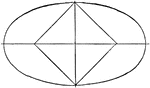### Parts of Ellipse

Diagram of an ellipse that can used to illustrate the different parts. Segment MN is the major axis,…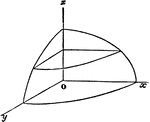### Ellipsoid

Each section is an ellipse. The surface is generated by an ellipse moving parallel to itself along two…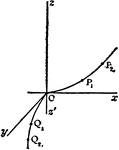### Hyperbolic Parabaloid

Three dimensional representation of a variable hyperbola moving parallel to itself along the parabolas…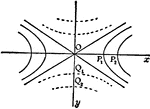### Hyperbolic Parabaloid

Two dimensional representation of variable hyperbola moving parallel to itself along the parabolas as…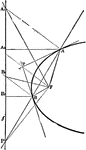### Parabola Foci Properties

The segments between the point of intersection of two tangents to a conic and their points of contact…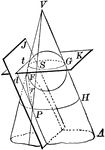### Cone Intersected by a Plane to Form a Parabola

Diagram of a cone intersected by plane J to form a parabola. Also pictured is a circle formed by the…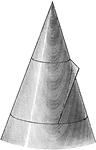### Conic Section Showing Parabola

Illustration showing the definition of a parabola as a conic section. "The section of a right circular…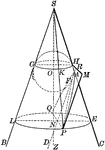### Conic Section Showing Parabola

Illustration showing the definition of a parabola as a conic section. "The section of a right circular…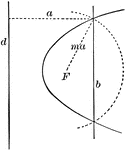### Construction of a Parabola

Diagram showing how to construct a parabola when given the directrix (d), the focus (F) and the eccentricity…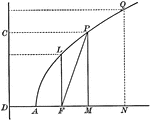### Parabola With Coordinates and Latus Rectum

Illustration of a parabola showing that any point of a parabola is the mean proportional between the…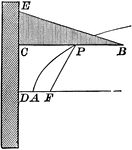### Parabola With Focus and Directrix

Illustration of a parabola - a curve which is the locus of a point that moves in a plane so that its…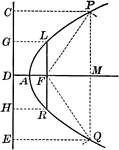### Parabola With Focus and Directrix

Illustration of a parabola - a curve which is the locus of a point that moves in a plane so that its…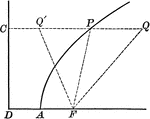### Point on Parabola

Illustration of a parabola showing point P is equidistant to the focus and the directrix.### Point on Parabola

Illustration of a parabola. "If a line PT is drawn from any point P of the curve, bisecting the angle…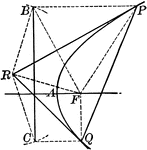### Tangent to a Parabola

Illustration of a tangent line drawn from an external point to a parabola.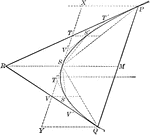### Tangents and Chords of a Parabola

Illustration showing that the area of a parabolic segment made by a chord is two thirds the area of…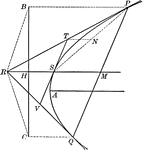### Tangents to a Parabola

Illustration showing that if two tangents RP and RQ are drawn from a point R to a parabola, the line…